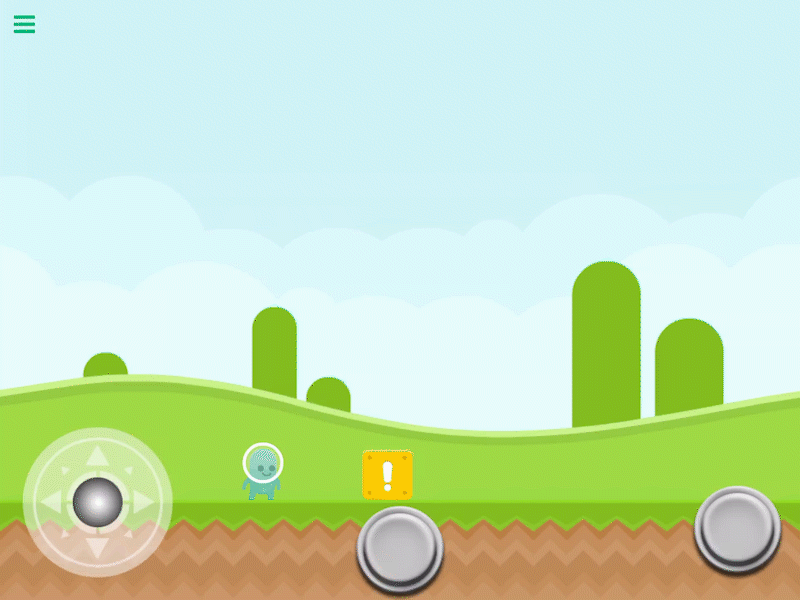# Apply Force

Description

This behavior will apply a force to any point on a single object.

This behavior will Trigger an event immediately.

Properties

 Object A This is the object you want to apply a force to. By default this is the current object selected. You can change this to any other object in your scene. Anchor This is the specific X and Y location on the object where the the force will be applied. Eg, the hand. on your character. You can enter the numbers manually, or visually by moving the blue target when selecting Object A from the behavior properties. Force X This is the amount of force to apply to the object along the X axis. this. Force is measured in newtons. Force Y This is the amount of force to apply to the object along the Y axis. this. Force is measured in newtons. Multiplier This will multiply the Force X and Force Y by the supplied number.

Outputs

 Object ID This outputs the Object ID.

Examples

You can apply a force of 10 newtons along the X axis of a box to make it slide to the right.